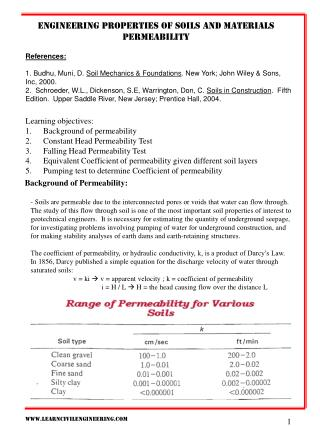DownloadDownload PresentationEngineering properties of soils and materials Permeability

# Engineering properties of soils and materials Permeability

Download Presentation## Engineering properties of soils and materials Permeability

- - - - - - - - - - - - - - - - - - - - - - - - - - - E N D - - - - - - - - - - - - - - - - - - - - - - - - - - -
##### Presentation Transcript

1. Engineering properties of soils and materials Permeability References: 1. Budhu, Muni, D. Soil Mechanics & Foundations. New York; John Wiley & Sons, Inc, 2000. 2. Schroeder, W.L., Dickenson, S.E, Warrington, Don, C. Soils in Construction. Fifth Edition. Upper Saddle River, New Jersey; Prentice Hall, 2004. • Learning objectives: • Background of permeability • Constant Head Permeability Test • Falling Head Permeability Test • Equivalent Coefficient of permeability given different soil layers • Pumping test to determine Coefficient of permeability Background of Permeability: - Soils are permeable due to the interconnected pores or voids that water can flow through. The study of this flow through soil is one of the most important soil properties of interest to geotechnical engineers. It is necessary for estimating the quantity of underground seepage, for investigating problems involving pumping of water for underground construction, and for making stability analyses of earth dams and earth-retaining structures. The coefficient of permeability, or hydraulic conductivity, k, is a product of Darcy’s Law. In 1856, Darcy published a simple equation for the discharge velocity of water through saturated soils: v = ki  v = apparent velocity ; k = coefficient of permeability i = H / L  H = the head causing flow over the distance L

2. Engineering properties of soils and materials Permeability Constant Head Test(ASTM D 2434) – Is used to determine the coefficient of permeability of coarse-grained soils. A typical constant head apparatus is shown below. Water is allowed to flow through the cylindrical sample of soil under a constant head (h). The outflow (Q) is collected in a graduated cylinder at a duration (t). ΔH = h and i = ΔH / L = h / L The flow rate through the soil is q = Q / t K = (Q * L) / (t * A * h) K = Coefficient of permeability Q = total quantity of water t = time L = Length of the coarse soil Temperature adjustment: The viscosity of the fluid, which is a function of temperature, influences the value of k. The experimental value (kToC) is corrected to a baseline temperature of 20oC using; k20oC = kToC (μToC / μ20oC) = kToC RT Where μ is the viscosity of water, T is the temperature in oC at which the measurement was made, and RT = μToC / μ20oC and is the temperature correction factor that can be calculated from; RT = 2.42 - .475 ln (T)

3. Engineering properties of soils and materials Permeability Falling-Head Test – Is used for fine-grained soil since the flow of water through these soils is too slow to get reasonable measurements from the constant head test. Water flows through the sample from a standpipe attached to the top of the cylinder. The head of water (h) changes with time as flow occurs through the soil. At different times the head of water is recorded. K = (a * L) / A(t2 – t1) ln (h1/h2) t = time L = Length of the fine soil A = cross section area of soil a= cross section area of tube K = Coefficient of permeability

4. Engineering properties of soils and materials Permeability Equivalent Coefficient of Permeability – In a stratified soil deposit where the k value in a given reaction changes from layer to layer, a equivalent Coefficient of Permeability (or called hydraulic conductivity) could be computed to simplify calculations. kH(eq) = 1/H (kH1H 1 + kH2H 2 + kH3H 3 + …+ kHnH n

5. Engineering properties of soils and materials Permeability Pumping Test to Determine the Coefficient of Permeability – A common method to determine the K value in the field is pumping water at a constant flow rate from a well and measuring the decrease in groundwater level at the observation wells. This test is only practical for coarse-grained soils K = q ln (r2/r1) / π (h22 – h21) q = flow rate of the pump r = distance from pumping well to observation wells h = height of water at the observation wells